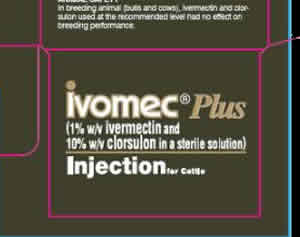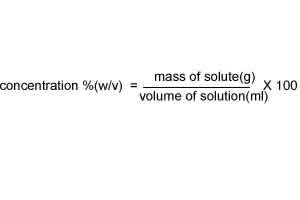Concentration(w/v) There are many ways to express the concentration of a solution. A bottle of medication has the label 1% (w/v) ivermectin. That means that in 100 ml of the solution there is 1 gram of ivermectin.. The label on the right states that the medication contains 10%(w/v) clorsulon where (w/v) is weight per volume. This is known as percentage mass/volume and gives the mass of the solute present in 100 ml of solution.120.0 ml of a medication contains 20.0 g of ethanol. What is the concentration (w/v) of ethanol in this solution? SolutionWhat volume of a 15.0% (w/v) alcohol solution will contain exactly 10.0 g of alcohol? Solution 200.0 ml of a 15.0% (w/v) hydrochloric acid solution will contain what mass of acid? Solution